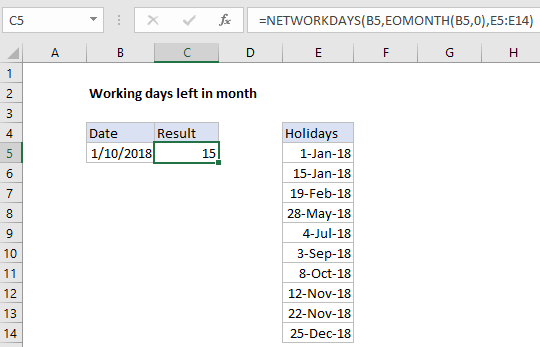## Excel Office

Excel How Tos, Tutorials, Tips & Tricks, Shortcuts

# How to calculate working days left in month in Excel

To calculate the number of workdays remaining in a month, you can use the NETWORKDAYS function. NETWORKDAYS automatically excludes weekends, and it can optionally exclude a custom list of holidays as well.

## Formula

`=NETWORKDAYS(date,EOMONTH(date,0),holidays)`## Explanation

In the example shown, the formula in C5 is:

`=NETWORKDAYS(B5,EOMONTH(B5,0),E5:E14)`

Were B5 contains a current date, and the range E5:E14 contains dates that are holidays.

Note: NETWORKDAYS includes both the start and end dates in the calculation if they are workdays.

Worked Example:   Get last weekday in month in Excel

### How this formula works

NETWORKDAYS is a built in function accepts a start date, end date, and (optionally) a range that contains holiday dates.

In this case, the start date is Jan 10, 2018, provided as cell B5. The end date is calculated using the EOMONTH function with an offset of zero, which returns the last day of the month of the date given. A list of holidays is provided as the range E5:E14.

Worked Example:   Sum by month in Excel

The EOMONTH function resolves to Jan 31, 2018, and the number of working days between Jan 10 and Jan 31 (inclusive) is 16. One of those working days (Jan 15) is listed as a holiday, so NETWORKDAYS returns a final result of 15.

Worked Example:   Get first day of month in Excel

### Custom workdays / weekends

To work with custom weekends (i.e. weekends are Sunday and Monday, etc.) switch to the more robust NETWORKDAYS.INTL function, which allows control over which days of the week are considered workdays.

### Working days remaining this month

To return the working days that remain in the current month, the formula can be adapted with the TODAY function as follows:

`=NETWORKDAYS(TODAY(),EOMONTH(TODAY(),0),holidays)`